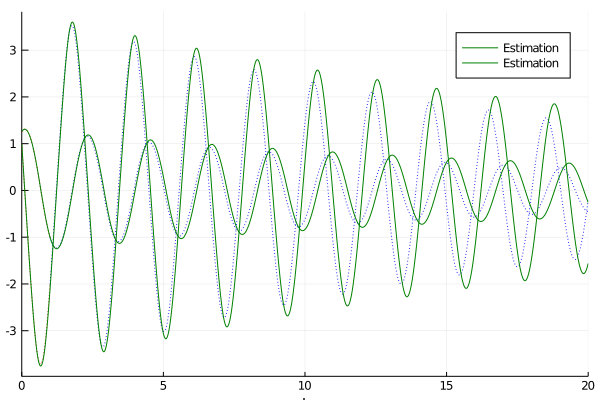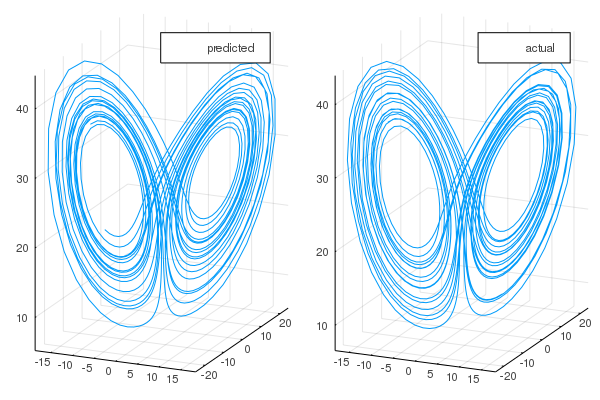﻿

# SciML Ecosystem Update: Automated Model Discovery with DataDrivenDiffEq.jl and ReservoirComputing.jl

You give us data and we give you back LaTeX for the differential equation system that generated the data. That may sound like the future, but the future is here. In this SciML ecosystem update I am pleased to announce that a lot of our data-driven modeling components are finally released with full documentation. Let's dive right in!

## DataDrivenDiffEq.jl: Dynamic Mode Decomposition and Sparse Identification of Models

DataDrivenDiffEq.jl has arrived, complete with documentation and a full set of examples. Thank Julius Martensen (@AlCap23) for really driving this effort. You can use this library to identify the sparse functional form of a differential equation via variants of the SInDy method given data and discover large linear ODEs on a basis of chosen observables through variants of dynamic mode decomposition. This library has many options for how the sparsification and optimization are performed to ensure it's robust, and integrates with ModelingToolkit.jl so that the trained basis functions work with symbolic libraries and have automatic LaTeXification via Latexify.jl. And, as demonstrated in the universal differential equations paper and highlighted in this presentation on generalized physics-informed learning, these techniques can also be mixed with DiffEqFlux.jl and neural networks to allow for pre-specifying known physics and discovering parts of models in a robust fashion.

As a demonstration, let's generate some data from a pendulum:

``````using DataDrivenDiffEq
using ModelingToolkit
using OrdinaryDiffEq
using LinearAlgebra
using Plots
gr()

function pendulum(u, p, t)
x = u
y = -9.81sin(u) - 0.1u
return [x;y]
end

u0 = [0.4π; 1.0]
tspan = (0.0, 20.0)
problem = ODEProblem(pendulum, u0, tspan)
solution = solve(problem, Tsit5(), atol = 1e-8, rtol = 1e-8, saveat = 0.001)

X = Array(solution)
DX = solution(solution.t, Val{1})``````

Let's automatically discover that differential equation from its timeseries. Now to perform SInDy, we define a set of basis functions via ModelingToolkit.jl:

``````@variables u[1:2]
h = Operation[u; u.^2; u.^3; sin.(u); cos.(u); 1]
basis = Basis(h, u)``````

Here we included a bunch of polynomials up to third order and some trigonometric functions. Now we tell SInDy what the timeseries data is and what the basis is and it'll spit out the differential equation system:

``````opt = SR3(3e-1, 1.0)
Ψ = SInDy(X[:, 1:1000], DX[:, 1:1000], basis, maxiter = 10000, opt = opt, normalize = true)``````
``````2 dimensional basis in ["u₁", "u₂"]
du₁ = p₁ * u₂
du₂ = sin(u₁) * p₃ + p₂ * u₂``````

And there you go: notice that it was able to find the right structural equations! `Ψ` is now of the form of the right differential equation, just from the data. We can then transform this back into DifferentialEquations.jl code to see how well we've identified the system and its coefficients:

``````sys = ODESystem(Ψ)
p = parameters(Ψ)

dudt = ODEFunction(sys)

estimator = ODEProblem(dudt, u0, tspan, p)
estimation = solve(estimator, Tsit5(), saveat = solution.t)``````We can now do things like, reoptimize the parameters with DiffEqParamEstim.jl or DiffEqFlux.jl, or look at the AIC/BIC of the fit, or etc.jl. See the DataDrivenDiffEq.jl documentation for more details on all that you can do. We hope that by directly incorporating this into the SciML ecosystem that it will become a standard part of the scientific modeling workflow and will continue to improve its methods.

## Automatic Discovery of Chaotic Systems via ReservoirComputing.jl

Traditional methods of neural differential equations do not do so well on chaotic systems, but the Echo State Network techniques in ReservoirComputing.jl do! Big thanks to @MartinuzziFrancesco who has been driving this effort. This library is able to train neural networks that learn attractor behavior and then predict the evolution of chaotic systems. More development will soon follow on this library as it was chosen to be one of the JuliaLang Google Summer of Code projects.## High Weak Order SDE Integrators

As part of our continued work on DifferentialEquations.jl we have added new stochastic differential equation integrators, `DRI1` and `RI1`, which are able to better estimate the expected value of the solution without requiring the computational overhead of getting high order strong convergence. This is only the start of a much larger project that we have accepted for JuliaLang's Google Summer of Code. Thank Frank Schafer (@frankschae) for driving this effort. He will be continuing to add methods for high weak convergence and fast methods for SDE adjoints to further improve DiffEqFlux.jl's neural stochastic differential equation support.

## Sundials 5 and LAPACK Integration

Sundials.jl now utilizes the latest version of Sundials, Sundials 5, for its calculations. Thanks to Jose Daniel Lara (@jd-lara) for driving this effort. Included with this update is the ability to use LAPACK/BLAS. This is not enabled by default because it's slower on small matrices, but if you're handling a large problem with Sundials, you can now do `CVODE_BDF(linear_solver=:LapackDense)` and boom now all of the linear algebra is multithreaded BLASy goodness.

Thanks to extensive maintanance efforts by Vaibhav Dixit (@Vaibhavdixit02), David Widmann (@devmotion), Kai Xu (@xukai92), Mohammad Tarek (@mohamed82008), and Rob Goedman (@goedman), the DiffEqBayes.jl library has received plenty of updates to utilize the most up-to-date versions of the Turing.jl, DynamicHMC.jl, and Stan probabilistic programming libraries (ModelingToolkit.jl automatically transforms Julia differential equation code to Stan). Together, this serves as a very good resource for non-Bayesian-inclined users to utilize Bayesian parameter estimation with just one function. See the parameter estimation documentation for more details.

As a quick update to the probabilistic programming space, we would like to note that the Turing.jl library performs exceptionally well in comparison to the other libraries. A lot of work had to be done in order to specifically find robustness issues in Stan and make the priors more constrained, while Turing.jl has had no issues. This has shown up in other places as well, where we have not been able to update our Bayesian Lorenz parameter estimation benchmarks due to robustness issues with Stan diverging Additionally, benchmarks on other ODE systems demonstrate a 5x and 3x performance advantage for Turing over Stan. Thus our examples showcase Turing.jl as being unequivically more robust for Bayesian parameter estimation of differential equation systems. We hope that, with the automatic differential equation conversion making testing between all of these libraries easy, we can easily track performance and robustness improvements to these probabilistic programming backends over time and ensure that users can continue to know and use the best tools for the job.

# Next Directions

Here's some things to look forward to:

• SuperLU_MT support in Sundials.jl

• The full release of ModelingToolkit.jl

• Automated matrix-free finite difference PDE operators

• High Strong Order Methods for Non-Commutative Noise SDEs

• Stochastic delay differential equations